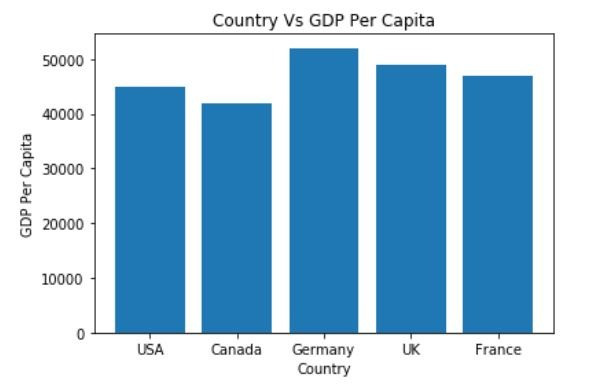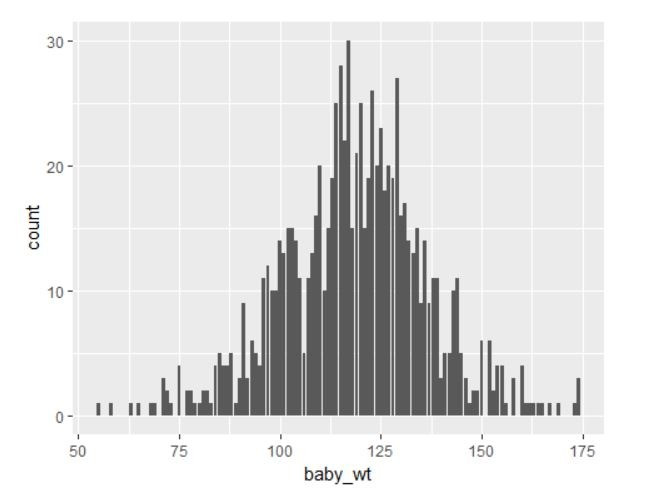Search

# Data Visualization Assignment Help In Machine Learning

There the different types of visualization techniques are used in machine learning, in this blog we will learn 5 top important visualization libraries that are mostly used by a data scientist to visualizing the data.

• Matplotlib

• Seaborn

• ggplot

• pyplot

• pygal

## Visualization with Matplotlib

Matplotlib is a free open source plotting library for creating static, animated, and interactive visualizations in Python.

It also supports the mathematics extension using NumPy.

### Installing matplotlib using "pip"

`pip install matplotlib`

After this need to import this using:

`import matplotlib.pyplot as plt`

Examples:

Plotting line graph using matplotlib: plot line using one variable(y coordinate):

```#plotting the line using one variable
import matplotlib.pyplot as plt
plt.plot([1, 2, 3, 4])
plt.show()

```Here the value of y is given and x is generated automatically and it starts from 0 with interval 0.5.

If both coordinates are given then:

`plt.plot([1, 2, 3, 4], [1, 4, 9, 16])`### Style formating

This is other arguments except for x, y, which used for styling the graph: "ro", "-b", etc

```plt.plot([1, 2, 3, 4], [1, 4, 9, 16], 'ro')
plt.axis([0, 6, 0, 20])
plt.show()```

Here "ro", means "red: o" shape, "-b", show "-" in blue color.### Histogram using matplotlib

You may use the below syntax to plot histogram using matplotlib.

Syntax:

```import matplotlib.pyplot as plt

x = [value1, value2, value3,....]
plt.hist(x, bins = number of bins)
plt.show()```

Example:

```import matplotlib.pyplot as plt

x = [1,1,2,3,3,5,7,8,9,10,
10,11,11,13,13,15,16,17,18,18,
18,19,20,21,21,23,24,24,25,25
]
plt.hist(x, bins=10)
plt.show()

```### Creating a Bar Chart Using Matplotlib:

Below the syntax which is used to creating the bar chart

```#ploting histogram using matplotlib
import matplotlib.pyplot as plt
plt.bar(xAxis,yAxis)
plt.title('title name')
plt.xlabel('xAxis name')
plt.ylabel('yAxis name')
plt.show()```

Example:

```import matplotlib.pyplot as plt

GDP_Per_Capita = [45000,42000,52000,49000,47000]

plt.bar(Country, GDP_Per_Capita)
plt.title('Country Vs GDP Per Capita')
plt.xlabel('Country')
plt.ylabel('GDP Per Capita')
plt.show()```## Visualization with Seaborn

Seaborn as a library is used in Data visualizations from the models built over the dataset to predict the outcome and analyse the variations in the data.

Seaborn Line Plots depict the relationship between continuous as well as categorical values in a continuous data point format.

first, need to install seaborn using pip:

`pip install seaborn`

then after import, it using:

`import seaborn`

Syntax to creating a line chart using seaborn:

`seaborn.lineplot(x, y, data)`

Where:

x: variable for the x-axis

y: variable for the y-axis

data: data values

Example:

```import pandas as pd
import seaborn as sns

Year = [1990, 1994, 1998, 2002, 2006, 2010, 2014]
Profit = [10, 62.02, 48.0, 75, 97.5, 25, 66.6]

data_plot = pd.DataFrame({"Year":Year, "Profit":Profit})

sns.lineplot(x = "Year", y = "Profit", data=data_plot)
plt.show()```you can also create more other visualization like bar, histogram, scatter plot, etc using seaborn.

## Visualization with "ggplot"

This is a visualization package that is used in R programming.

Let suppose data is like:

##   baby_wt  income mother_age     smoke gestation mother_wt ## 1     120 level_1         27 nonsmoker       284       100 ## 2     113 level_4         33 nonsmoker       282       135 ## 3     128 level_2         28    smoker       279       115 ## 4     108 level_1         23    smoker       282       125 ## 5     132 level_2         23 nonsmoker       245       140 ## 6     120 level_2         25 nonsmoker       289       125

#### Plot1: Simple Bar-plot (Showing distribution of baby’s weight)

`ggplot(data = Birth_weight,aes(x=baby_wt))+geom_bar()`

The above code has three parts:

• data: It is the name of the data-set

• aes: This is where we provide the aesthetics, i.e. the “x-scale” which will be showing the distribution of “baby_wt”(baby weight)

• geometry: The geometry which we are using is bar plot and it can be invoked by using geom_bar() function.#### Plot2: Simple Bar-plot (Showing distribution of mother’s age)

`ggplot(data = Birth_weight,aes(x=mother_age))+geom_bar()`

## Visualization Using "Pygal"

Pygal specializes in allowing the user to create SVGs. Besides the scalability of an SVG, you can edit them in any editor and print them in very high-quality resolution.

Installing "pygal" using "pip"

`pip install Pygal`

Import "pygal"

`import pygal`

Creating the variable to create the graph:

```import pygal
bar_chart=pygal.Bar()()```

Adding some values like "title", etc.

```import pygal
bar_chart = pygal.Bar()
bar_chart.title = " ratio"```

Example:

```import pygal
bar_chart = pygal.Bar()
bar_chart.title = "Ratio"
bar_chart.render_in_browser()```where render_in_browser() is used to render the graph in the web - browser.

Contact us to get help related to data visualization in machine learning using below contact details: contact@codersarts.com